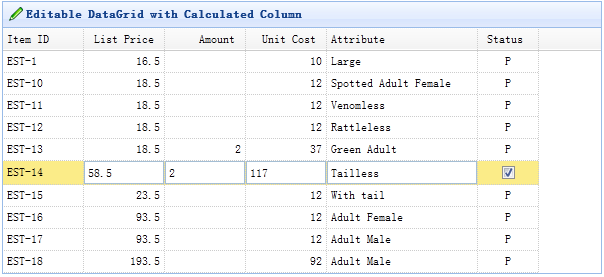# Calculate two column values in editing DataGrid

Tutorial » Calculate two column values in editing DataGrid

In this tutorial you will learn how to include a calculated column in a editing datagrid. A calculated column generally contains some value calculated from one or more other columns.First, create the editable datagrid. Here we create some editable columns, the 'listprice','amount' and 'unitcost' columns are defined as numberbox editing type. The calculated column is 'unitcost' field that will be the result of multiplying listprice times amount.

Now we begin an editing action when user click a row.